# Center of Torsion of a Thin U-BeamThe purpose of this test is to take into account the center of torsion.
The type of test is a 1D model, non symmetric section with center of torsion, different from the center of gravity. Both predefine sections (Thin U-Beam) and define from a surface sections (Beam from surface) are used.Reference:

Modélisation des structures par éléments finis, BATOZ J.L., DHATT G., Vol.2; Hermès Edition, Paris 1990.

##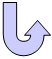Specifications

### Geometry Specifications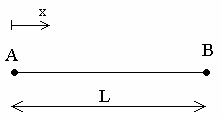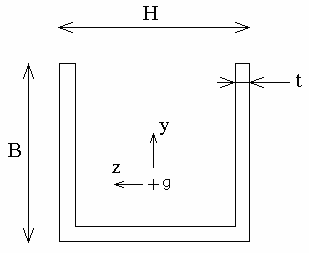L = 0.1 m  H = 0.01 m  B = 0.008 m  t = 0.0004 m

### Analysis Specifications

 Young Modulus (material): E = 200 GPa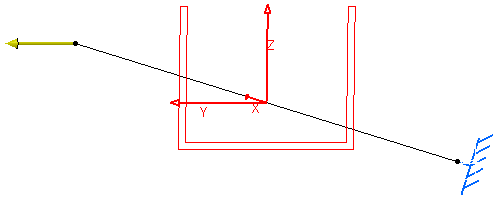Poisson's Ratio (material): ν = 0.266 Mesh Specifications: One beam element on the whole length Mesh size: 100 mm Restraints: Clamp on A Loads (Distributed force): On B: Pz = 1N

##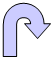Results

### Analytical Solution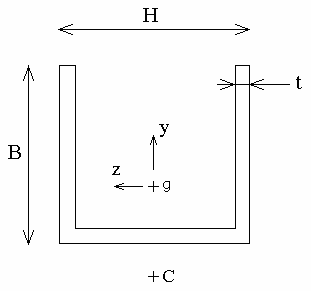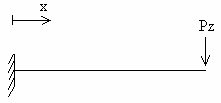• g: center of gravity
• C: center of torsion/shearing. C is the point of the section where shearing restraints due to a cutting effort generate a null moment of torsion.
The rotation of the section on x is given by: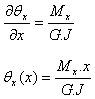where: G: shearing coefficient (Pa) For an isotropic material: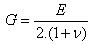J: Inertia/Constant of torsion (m4) For a U-Beam section (valid formula for t < B and H):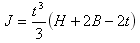Mx: moment of torsion (N.m)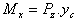yc: coordinates of point C on y (the origin of the axis system is the center of gravity) For a U-Beam section: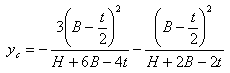For:
J = 0.5376 x 10-12 m4
yc = -5.65046 x 10-3 m
x = L

The result of the analytic solution is: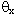= -1.33063 x 10-2 rad

### Computed results

 Results Reference Case 1 "Thin U-beam" Case 2 "Beam from surface"(rad) -1.33063 x 10-2 -1.33063 x 10-2 -1.35608 x 10-2 Normalized results 1 1 1.019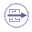To Perform the Test:

The thin_u_beam.CATAnalysis document presents a complete analysis of this case.

To compute the case, proceed as follow:

1. Open the CATAnalysis document.

2. Compute the case in the Generative Structural Analysis workbench.

3. Create a local sensor (Rotation vector).